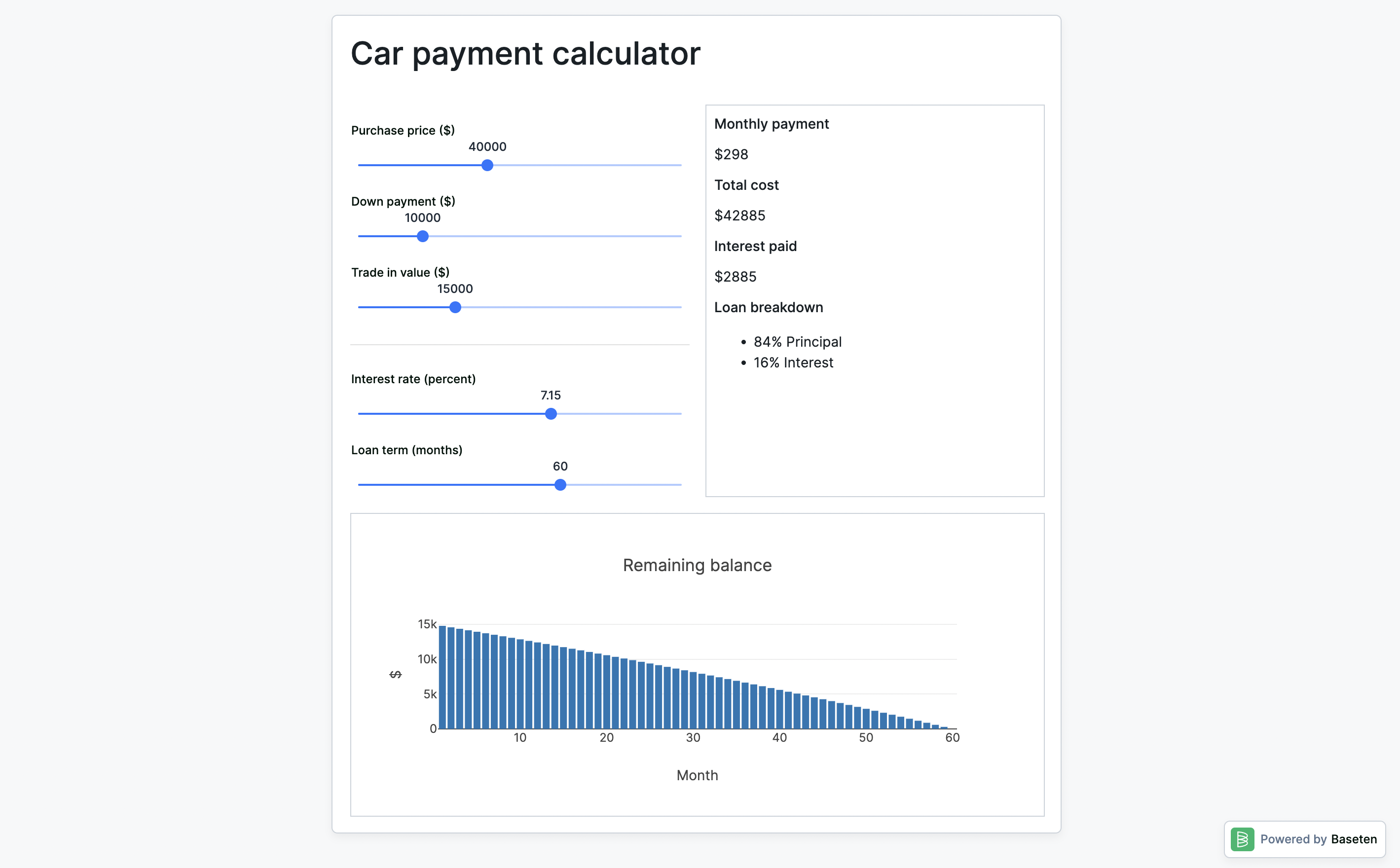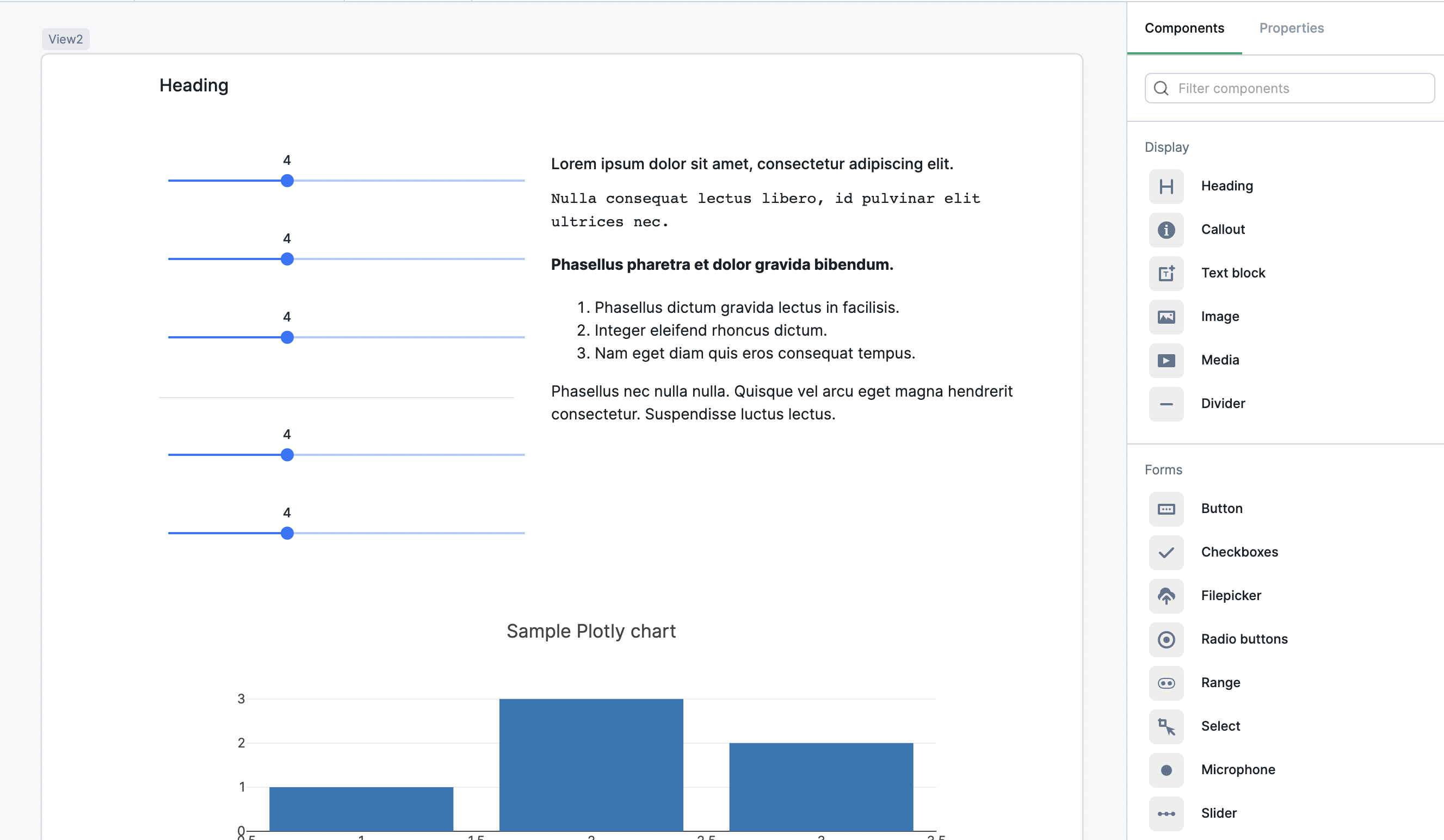# Stateful UI with Python

Use Python in the view builder to create a stateful user interface
Building a stateful, interactive web application in Baseten's view builder doesn't require learning JavaScript, HTML, or CSS. Instead, use drag-and-drop components and run Python directly in the web browser to build apps.The finished application
In this tutorial, we'll build an auto loan calculator. Users can input the purchase price, down payment, and trade in value for a car purchase, then see how interest rate and loan term affect monthly payoff and total cost. You can try out the finished app here.

### Step 1: Building the UI

If this tutorial was a recipe for baking delicious cookies, this is where I would list the ingredients needed and the quantities. Well, maybe I will anyway!
Ingredients:
Arrange your components on the page with the layout below:The application layout
Then, configure these components properties individually. To configure a component, click on it and use the "Properties" menu on the right side of the screen.
Start by setting the heading to something descriptive like "Car payment calculator." For the text block and Plotly chart components, turn on the "Show border" toggle but otherwise you can leave them as-is. We'll configure them at the end of the tutorial.
As for the sliders, these require more attention. For each slider, you'll need to rename the component and set its various properties. Rename the component by clicking on the default name, like `slider1`, in the Properties menu above the display properties. Set the component properties by filling in the relevant fields.
Configure the components as follows:
• Name: `purchase_price_slider`
• Label: `Purchase price (\$)`
• Minimum value: `0`
• Maximum value: `100000`
• Initial value: `0` (we'll configure this later)
• Increment: `1000`
• Name: `down_payment_slider`
• Label: `Down payment (\$)`
• Minimum value: `0`
• Maximum value: `50000`
• Initial value: `0` (we'll configure this later)
• Increment: `1000`
• Name: `trade_in_slider`
• Label: `Trade in value (\$)`
• Minimum value: `0`
• Maximum value: `50000`
• Initial value: `0` (we'll configure this later)
• Increment: `1000`
• Name: `interest_rate_slider`
• Label: `Interest rate (percent)`
• Minimum value: `0`
• Maximum value:
• Initial value: `0` (we'll configure this later)
• Increment: `.05`
• Name: `loan_term_slider`
• Label: `Loan term (months)`
• Minimum value: `0`
• Maximum value: `96`
• Initial value: `0` (we'll configure this later)
• Increment: `6`
With your sliders configured, let's build the engine behind this user interface.

Now we give our application state. We want this state to exist from the moment a user loads the page. Select the page canvas as a component by clicking the grey tag just above the upper left corner of the canvas.
Under the "Event handlers" header, click "Add event handler" to create an on-load event handler. We'll make five of these, each of which sets state. Set state as follows:
• `purchase_price`: 40000
• `down_payment`: 10000
• `trade_in_value`: 15000
• `interest_rate`: 7.15
• `loan_term_months`: 60
Once these five event handlers are configured to set state, reload the page and the state will appear with the correct values. You can see them by clicking the Data explorer on the lower-right corner of the screen.
Now, we will set the initial values of our five sliders to the appropriate state field. You can do this with a binding. For example, click on the "Purchase price" slider and find the "Initial value" field in the Properties menu. Type `{{` into that field to pull up a list of bindings. Type in `purchase_price` and use "Tab" to complete the binding.
Repeat this for each of the other four sliders.

### Step 3: Writing the Python function

To write a Python function, open the Data explorer in the lower-right corner of the screen. Click the "Create" link next to the "Functions" header.
Name your function `calculate_loan` in the grey field above the language selector.
Before we write code, let's pass parameters into the function. We'll give it the values of each of the five sliders. To pass in these bindings, type `{{` and options will appear. Keep typing until you find the one you want, then press "Tab" to complete the binding.
Set the parameters as follows (remember, we are using slider component values, not state values):
• `price`: `{{purchase_price_slider.value}}`
• `down`: `{{down_payment_slider.value}}`
• `trade`: `{{trade_in_slider.value}}`
• `rate`: `{{interest_rate_slider.value}}`
• `term`: `{{loan_term_slider.value}}`
Now, we code! Click the expand icon in the upper-right corner of the code box to open a larger code editor. Paste the following:
# Convert interest from annual to monthly
monthly_rate = rate/1200
# Get a list of months for the plotly chart
months = [x for x in range(1, term + 1)]
# Determine loan amount
loan = price - (down + trade)
# Use formula to calculate monthly payment
payment = (loan * monthly_rate) / (1 - (1 + monthly_rate)**(-1*term))
# Calculate total amount paid
total_payment = round(payment*term)
# Calculate how much of the payment went to interest
interest = total_payment - loan
# Calculate total cost
total_cost = price + interest
# Calculate percent of payment to principal
percent_principal = round((loan / total_payment)*100)
# Calculate percent of payment to interest
percent_interest = 100 - percent_principal
# Calculate loan balance per month for plotly chart
payoff = []
for i in range(term):
loan = max((loan*(1+monthly_rate)) - payment, 0)
payoff.append(loan)
# Return all calculated values & format plotly data
return {
"chart": str({
"data":[
{"type":"bar",
"x":months,
"y":payoff
}],
"layout":
{"title":{"text":"Remaining balance"},
"xaxis":{"title":"Month"},
"yaxis":{"title":"\$"}}
}),
"payment": round(payment),
"cost": total_cost,
"interest": interest,
"pprincipal": percent_principal,
"pinterest": percent_interest
}
Let's break down what's going on in this function. The code is commented line-by-line, but broadly it works in three steps:
1. 1.
Calculate relevant values for monthly payment, total payment, total interest, etc using common formulas
2. 2.
Calculate the monthly balance assuming the standard payment is applied
3. 3.
Return all calculated values. Monthly balance is used for the plotly chart, while the other values are used directly in the text block.
Again, this Python code runs directly in the user's browser with no back-end calls required.

### Step 4: Displaying function output

The last step is wiring up the function's output throughout the view. We are using the returned data in two places: the text block and the plotly chart.
Our function returns a dictionary. We can reference an individual value from that dictionary with a binding to `{{function_name.key}}`.
Here's what the markdown I used in the text block looks like:
**Monthly payment**
\${{calculate_loan.payment}}
**Total cost**
\${{calculate_loan.cost}}
**Interest paid**
\${{calculate_loan.interest}}
**Loan breakdown**
* {{calculate_loan.pprincipal}}% Principal
* {{calculate_loan.pinterest}}% Interest
Finally, we need to configure the Plotly chart. Thanks to our work in step 3 to make the data well-formatted, all you need to do is add a binding to `{{calculate_loan.chart}}` in the Plotly chart component's "Chart data" field.
The app is complete! Experiment with different values on the slider and watch the output adjust in real time.